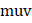# A particle of massis moving horizontally with a constant velocitytowards a rigid wall that is moving in opposite direction with a constant speed  . Assuming elastic impact between the particle and wall, the work done by the wall in reflecting the particle is equal to a) (1/2)b) (1/2)c) (1/2)d) None of these

## Question ID - 100239 :- A particle of massis moving horizontally with a constant velocitytowards a rigid wall that is moving in opposite direction with a constant speed  . Assuming elastic impact between the particle and wall, the work done by the wall in reflecting the particle is equal to a) (1/2)b) (1/2)c) (1/2)d) None of these

3537

(d)Next Question :
 A particle of mass 4is projected from the ground at some angle with horizontal. Its horizontal range isAt the highest point of its path it breaks into two pieces of massesand 3respectively, such that the smaller mass comes to rest. The larger mass finally falls at a distancefrom the point of projection, whereis equal to a)b)c)d) None of these